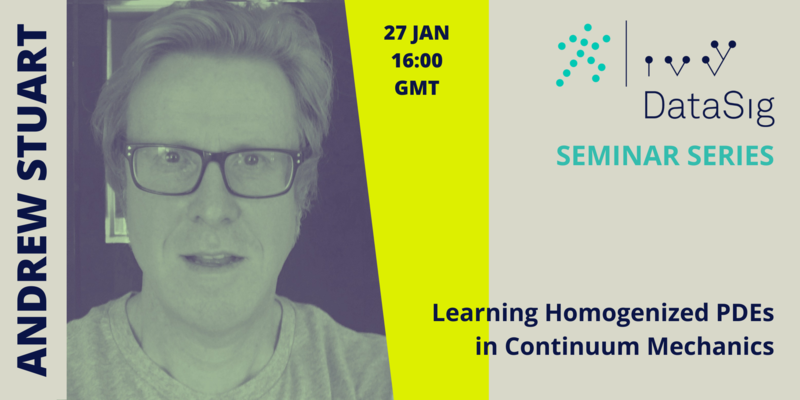# Andrew Stuart### Abstract

Neural networks have shown great success at learning function approximators between spaces X and Y, in the setting where X is a finite dimensional Euclidean space and where Y is either a finite dimensional Euclidean space (regression) or a set of finite cardinality (classification); the neural networks learn the approximator from N data pairs {x_n, y_n}. In many problems arising in the physical and engineering sciences it is desirable to generalize this setting to learn operators between spaces of functions X and Y. The talk will overview recent work in this context.

Then the talk will focus on work aimed at addressing the problem of learning operators which define the constitutive model characterizing the macroscopic behaviour of multiscale materials arising in material modeling. Mathematically this corresponds to using machine learning to determine appropriate homogenized equations, using data generated at the microscopic scale. Applications to visco-elasticity and crystal-plasticity are given.

### Our speaker

Andrew Stuart has research interests in applied and computational mathematics, and is interested in particular in the question of how to optimally combine complex mechanistic models with data. He joined Caltech in 2016 as Bren Professor of Computing and Mathematical Sciences, after 17 years as Professor of Mathematics at the University of Warwick (1999 to 2016). Prior to that he was on the faculty in The Departments of Computer Science and Mechanical Engineering at Stanford University (1992 to 1999), and in the Mathematics Department at Bath University (1989 to 1992). He obtained his PhD from the Oxford University Computing Laboratory in 1986, and held postdoctoral positions in Mathematics at Oxford University and at MIT in the period 1986 to 1989.